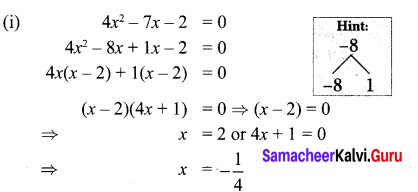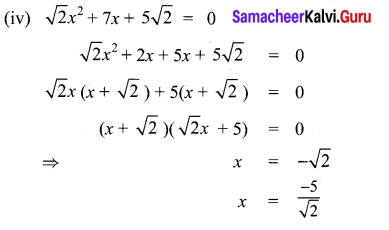You can Download Samacheer Kalvi 10th Maths Book Solutions Guide Pdf, Tamilnadu State Board help you to revise the complete Syllabus and score more marks in your examinations.

## Tamilnadu Samacheer Kalvi 10th Maths Solutions Chapter 3 Algebra Ex 3.10

10th Maths Exercise 3.10 Samacheer Kalvi Question 1.
Solve the following quadratic equations by factorization method
(i) 4x2 – 7x – 2 = 0
(ii) 3(p2 – 6) = p(p + 5)
(iii) $$\sqrt{a(a-7)}=3 \sqrt{2}$$
(iv) $$\sqrt{2} x^{2}+7 x+5 \sqrt{2}=0$$
(v) 2x2 – x + $$\frac{1}{8}$$ = 0
Solution:(ii) 3(p2 – 6) = p(p + 5)
3p2 – 18 = p2 + 5p ⇒ 392 – 5p – 18 = 0
⇒ 2p2 – 5p – 18 = 0
⇒ (2p – 9) (p + 2) = 0 ⇒ p = $$\frac{9}{2}$$, -2

(iii) $$\sqrt{a(a-7)}=3 \sqrt{2}$$
Squaring on both sides
a(a – 7) = 9 × 2
a2 – 7a – 18 = 0
a2 – 9a + 2a – 18 = 0
a(a – 9) + 2(a – 9) = 0
(a – 9) (a + 2) = 0
⇒ a = 9, a = -2(v) 2x2 – x + $$\frac{1}{8}$$ = 0
16x2 – 8x + 1 = 0
16x2 – 4x – 4x + 1 = 0
4x(4x – 1) – 1(4x – 1) = 0
(4x – 1) (4x – 1) = 0
⇒ x = $$\frac{1}{4}, \frac{1}{4}$$

Ex 3.10 Class 10 Samacheer Question 2.
The number of volleyball games that must be scheduled in a league with n teams is given by G(n) = $$\frac{n^{2}-n}{2}$$ where each team plays with every other team exactly once. A league schedules 15 games. How many teams are in the league?
G(n) = $$\frac{n^{2}-n}{2}$$
$$\frac{n^{2}-n}{2}$$ = 15